Here we are providing Class 11 chemistry Important Extra Questions and Answers Chapter 4 Chemical Bonding and Molecular Structure. Chemistry Class 11 Important Questions are the best resource for students which helps in Class 11 board exams.

## Class 11 Chemistry Chapter 4 Important Extra Questions Chemical Bonding and Molecular Structure

### Chemical Bonding and Molecular Structure Important Extra Questions Very Short Answer Type

Question 1.
What change in energy takes place when a molecule is formed from its atoms?
There is a fall in energy.

Question 2.
Arrange the following in order of increasing bond strengths.
F2, O2, N2, Cl2
F2 < Cl2 < O2 < N2

Question 3.
Name the shapes of the following molecules: CH4, C2H2, CO2.
CH4: Tetrahedral; C2H2: Cylindrical; CO2: linear

Question 4.
Arrange the following in order of increasing strengths of hydrogen bonding O, F, S, Cl, N.
Cl < S < N < O < F;

Question 5.
Identify the compound/compounds in the following in which S does not obey the Octet rule: SO2, SF2, SF4, SF6.
SF4, SF6.

Question 6.
Name one compound each involving sp3, sp2, sp hybridization.
sp3: CH4: sp2: C2H4: sp: C2H2

Question 7.
Which orbitals can overlap to form a cr-bond and which orbitals can do so to form a π bond?
s-s, s-p, p-p form a bond, and only p-p form π bond.

Question 8.
Which electrons take part in bond formation?
Valence electrons present in the outermost shell.

Question 9.
What are SI units of dipole moment?
Coulomb meter (Cm).

Question 10.
Name the method generally used for the calculation of lattice energy or electron affinity.
Borh-Haber cycle.

Question 11.
You are given the electronic configuration of five neutral atoms A, B, C, D, and E.
A – 1s2, 2s2 2p6 3s2;
B = 1s2, 2s2 2p6, 3s1
C = 1s2, 2s2 2p1;
D = 1s2, 2s2 2p5,
E = 1s2, 2s2 2p6.
Write the empirical formula for the substance containing
(i) A and D,

(ii) B and D
BD

(iii) only D
D2

(iv) Only E.
E.

Question 12.
Which type of forces holds the atoms together in an ionic compound?
Electrostatic forces of attraction.

Question 13.
Choose the compounds containing ionic, covalent, and coordinate bonds out of the following: MgO, CH4, CaCl2, HCl,
NH+4,O3.
IONIC: MgO, CaCl2;
Covalent: CH4, HCl;
Coordinate:NH+4, O3.

Question 14.
Write the Lewis structure of the polyatomic ions CN, SO42-.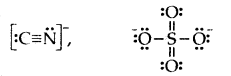Question 15.
Arrange the following in order of decreasing C-C bond length:
C2H6, C2H2, C2H4
H3C – CH3 > H2C = CH2 > HC ≡ CH.

Question 16.
Arrange the following in order of decreasing boiling point.
H2O, H2S, H2Se.
H2O > H2S > H2Se.

Question 17.
Which of the following has the maximum bond angle? Why?
H2O, CO2, NH3 CH4.
CO2. Because it is a linear molecule (bond angle is 180°).

Question 18.
Write down the resonance structures of nitrous oxide.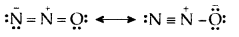Question 19.
Sodium metal vaporizes on heating and the vapors have diatomic molecules of sodium (Na2). What type of bonding is present in these molecules?
Covalent.

Question 20.
Predict the molecular geometries of (a) CIF3 (b) ICI4
(a) T-shaped
(b) Square planar.

Question 21.
What kind of bond exists between two non-metallic elements?
Covalent bonds because the difference of electronegativity between two non-metals is usually very small.

Question 22.
Which of the following bonds is most polar?
S – Cl, S – Br, Se – Cl, or Se – Br.
Se – Cl.

Question 23.
Write the Lewis structure of PCl3.Question 24.
Define valency.
The number of electrons that an atom gains or loses or shares with other atoms to attain noble gas configuration is termed its valency.

Question 25.
Explain why O-O bond lengths in ozone molecule are equal?
O-O bond lengths in ozone molecules are equal because of resonance between their structures.

Question 26.
MgCl2 is linear, but SnCl2 is angular. Why?
MgCl2 has sp hybridization, whereas SnCl2 has sp2 hybridization.

Question 27.
Why CCl4 does not give white precipitates with silver nitrate?
CCl4 is a covalent molecule and does not give Cl ions in solution.

Question 28.
What is the approximate bond strength for an ionic bond?
It is about 400 kJ mol-1

Question 29.
What is the approximate bond strength for a covalent bond?
It is about 100 kJ mol-1.

Question 30.
Though chlorine has nearly the same electronegativity as nitrogen, yet there is no hydrogen bonding in HCl. Why?
The size of the chlorine atom (3 orbits) is bigger than N (2 orbits).

Question 31.
Which will form a stronger ionic bond?
(a) Na and F
(b) Na and Cl.
(a) Na and F.

Question 32.
Out of MgO and NaCl which has more lattice energy?
MgO.

Question 33.
Draw Lewis structure of N3- ion.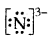Question 34.
On what factors the polarity of the bond depends?
Difference of electronegativity of the two atoms.

Question 35.
Arrange NaCl, AlCl3 MgCl2 in increasing covalent character.
NaCl < MgCl2 < AlCl3.

Question 36.
Arrange KCl, LiCl, NaCl in increasing ionic character.
LiCl < NaCl < KCl.

Question 37.
BF3 is non-polar. Why?
The dipole moment of BF3 is zero because the BF3 molecule is symmetrical.

Question 38.
What type of bond is formed when atoms have
(i) zero difference of electronegativity
Non-polar covalent

(ii) a little difference of electronegativity
Polar covalent

(iii) high difference of electronegativity?
Electrovalent.

Question 39.
What is the state of hybridization of C atoms in
(i) diamond
It is sp3 in diamond

(ii) graphite?
It is sp2 in graphite.

Question 40.
What type of orbitals can overlap to form a covalent molecule?
Half-filled atomic orbitals containing electrons with opposite spins.

Question 41.
Why glucose, fructose, sucrose, etc. are soluble in the water though they are covalent compounds?
These compounds contain OH groups which can form H- bonds with water.

Question 42.
What is the shape of NH4+ ion?
Due to the sp3 hybridization of N in NH4+, it is tetrahedral in shape.

Question 43.
Both FIF and H2O are associated through hydrogen¬bonding, yet the boiling point of HF is lower than that of HzO. Why?
H-bonds formed by H2O are double in number than those formed by HF molecules.

Question 44.
Why ethyl alcohol is completely miscible with water?
This is because the OH group present in ethyl alcohol forms H-bonds with water.

Question 45.
Benzene ring contains alternate single and double bonds, yet all the C-C bonds are of equal length. Why?
This is due to resonance in benzene.

Question 46.
When is a molecule paramagnetic in nature?
A molecule is paramagnetic when it contains half-filled molecular orbitals.

Question 47.
Arrange the following molecular species in increasing order of stability.
N22- < N2- = N2+ < N2

Question 48.
Explain on the basis of the molecular orbital diagram why O2 should be paramagnetic?
O2 molecule contains one unpaired electron in each of one π2px and π2py orbitals.

Question 49.
Define antibonding molecular orbital.
The molecular orbital formed by the subtractive effect of the electron waves of the combining atomic orbitals is called an antibonding molecular orbital.

Question 50.
What is the effect of the process C2 → C2+ + e on bond order of C2? [A.IS.B. 2002]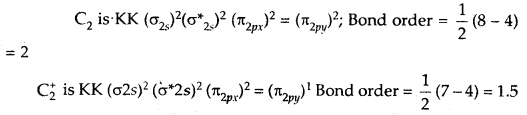### Chemical Bonding and Molecular Structure Important Extra Questions Short Answer Type

Question 1.
Which out of CH3F and CH3Cl has a higher dipole moment and why?
The dipole moment of CH3Cl is greater than that of CH3F. The C-F bond length in CH3F is smaller than the C-Cl bond length in CH3Cl. The charge separation in the C-F bond is more than in the Cl-C bond- fluoride being more electronegative than chlorine. The bond length has a greater effect than the charge separation. Hence the dipole moment of CH3C1 is greater than that of CH3F.

Question 2.
Define the term chemical bond. What are its different types?
The attractive forces which hold the constituent atoms in molecules or species in lattices etc. are called a chemical bond.

They are of the following types:

1. Electrovalent or ionic bond
2. Covalent bond
3. Coordinate or dative bond
4. metallic bond
5. hydrogen bond
6. van der Waals forces.

Question 3.
Why covalent bonds are called directional bonds whereas ionic bonds are called non-directional?
A covalent bond is formed by the overlap of half-filled atomic orbitals which have definite directions. Hence covalent bond is directional. In ionic compounds, each ion is surrounded by a number of oppositely charged ions and hence there is no definite direction.

Question 4.
AlF3 is a high melting solid whereas SiF4 is a gas. Explain why?
AlF3 is an ionic solid due to the large difference in electronegativities of Al and F whereas SiF4 is a covalent compound and hence there are only weak van der Waal’s forces among its molecules.

Question 5.
Using the VSEPR theory identifies the type of hybridization and draw the structure of OF2 What are oxidation states of O and F?
The electron dot structure of OF2 is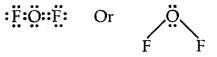Thus the central atom (O-atom) has 4 pairs of electrons (2 bond pairs and 2 lone pairs). Hence oxygen in OF2 is sp3 hybridised and the molecule is v-shaped oxidation state of F = – 1, oxidation state of O = + 2.

Question 6.
Account for the following: The experimentally determined N-F bond length in NF3 is greater than the sum of the single covalent radii of N and F.
This is because both N and F are small and hence have high-electron density. So they repel the bond pairs thereby making the N-F bond length larger.

Question 7.
Explain why the bond angle of NH3 is greater than that of NF3 while the bond angle of PH3 is less than that of. PF3.
Both NH3 and NF3 are pyramidal in shape with one lone pair on N. However as F has a higher electronegativity than H, the electron pair is attracted more towards F in NF3, i.e., the bond pairs of electrons are away from N or in other words, the distance between bond pairs is more. Hence the repulsions between bond pairs in NF3 are less than in NH3. Thus the lone pair repels the bond pairs of NF3 more than it does in NH3 As a result the bond angles decrease to 102.4° whereas, in ammonia, it decreases to 107.5° only.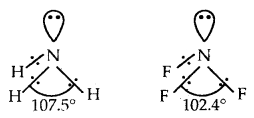PH3 and PF3 are also pyramidal in shape with one lone pair of P. But PF3 has a greater bond angle than PH3 (opposite to NH3 and NF3). This is due to resonance in PF3 leading to the partial double bond character as shown below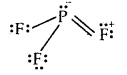As a result, repulsions between P-F bonds are larger and hence the bond angle is large. There is no possibility for the formation of double bonds in PH3.

Question 8.
Why HCl is polar whereas the Cl2 molecule is non-polar?
In Cl2 both atoms have the same electronegativity. Hence the shared pair of electrons is attracted equally by both and remains exactly in the center. NO end acquires a negative or positive charge. In HCl, chlorine is more electronegative than H. Hence shared pair of electrons is more attracted towards chlorine, which, therefore acquires a negative charge while H acquires a positive charge.

Question 9.
Write the Lewis structure of the nitrate ion NO2
Total no. of valence electrons on N = 2s22p3 = 5
Total no. of valence electrons on two oxygen atoms = 2s22p4
= 2 × 6 = 12
add one electron for negative charge = 5 + 12 + 1 = 18.
The skeletal structure of NO2 is written as O N O

Draw a single bond (one shared electron pair) between the nitrogen atom and each of the two oxygen atoms completing the octets on oxygen atoms. This, however, does not complete the octet on Nitrogen if the remaining two electrons, constitute lone pair on it.Hence we have to resort to multiple bonding between N and one of the oxygen atoms (in this ease a double bond). This leads to the following Lewis dot structures.Question 10.
Draw the Lewis dot structure of CO3 ion.
Total no. of valence electrons of CO3 = 4 + 3 × 6 = 22
total no. of valence electrons on CO3 = 22 + 2 = 24
The skeletal structure of CO3 is
Putting one shared pair of electrons between C and O and completing the Octets of oxygen, we have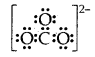In the above structure, an octet of oxygen is not complete. Hence multiple bonding is required between C and O.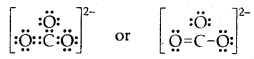Question 11.
Calculate the formal charge on each oxygen atom of O3 molecules and write its structure with formal charges.
Lewis structure of O3 is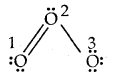Formal charge on O1 = 6 – 4 – $$\frac{1}{2}$$(4) = 0
Formal charge on 0(2) = 6 – 2 – $$\frac{1}{2}$$ (6) = + 1
Formal charge on 0(3) = 6 – 6 – $$\frac{1}{2}$$ (2) = – 1.

Hence we represent O3 along with formal charges as follows.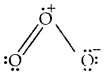Question 12.
Describe briefly the limitations of the Octet rule.

1. In some molecules like LiCl, BeCl2, BF3, AlCl3, central atoms like Li, Be, B, Al have not completed their octets Li: Cl,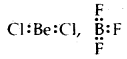2. In some molecules, there are an odd number of electrons like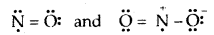3. In some molecules like PF5, SF6, IF7, the central atoms like P, S, I have 10,12,14 electrons respectively.
4. Some noble gases also combine with oxygen and F to form a no. of compounds like XeOF2, XeF2, XeF6, etc.
5. Octet theory does not explain the shape of the molecules.
6. It does not explain the relative stability of the molecules being totally silent about the energy of a molecule.

Question 13.
Explain the structure of the CO2 molecule on the basis of resonance.
The experimentally determined C to O bond length in CO2 is 115 pm. The lengths of a normal C to oxygen double bond (C = 0) and carbon, to oxygen triple bond (C ≡ O), are 121 pm and 110 pm respectively. The C-O bond lengths in CO2 (115 pm) lie between the values of C =0 and C-O. Obviously, a single Lewis structure cannot depict this position and it becomes necessary to write more than one Lewis structure.

∴ CO2 is best described as the resonance hybrid of the canonical or resonance forms I, II, and IIIQuestion 14.
How would the bond lengths vary in the dicarbon species
C2, C2, C22-?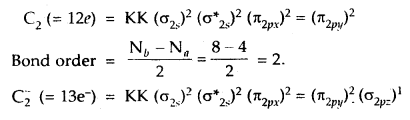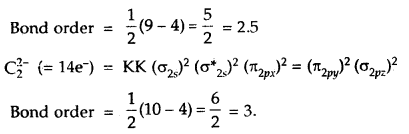As bond lengths are inversely proportional to bond order
∴ Bond lengths will be in order.
C2 > C2 > C22-.

Question 15.
What is the effect of the following ionization process on the bond order of O2?
O2 → O2+ + e
O2 → O2 + eThus bond order of O2 which is = 2
increases to 2.5 in O2+.

Question 16.
Draw the Lewis dot diagram of nitric acid, sulphuric acid, phosphorus acid, and hypophosphorous acid.Question 17.
Out of sigma and pi bonds, which one is stronger and why?
Sigma (a) bond is stronger This is because a bond is formed by head-on overlapping of atomic orbitals and such overlapping being on the internuclear axis is large, n bond is formed by sidewise overlapping which is small and so a Pi bond is weaker.

Question 18. Arrange the following in order of decreasing bond angles
(i) CH4, NH3 H2O, BF3, C2H2
C2H2 (180°) > BF3 (120°) > CH4 (109°, 28′) > NH3 (107°) > H2O (104.5°)

(ii) NH3 NH2, NH4
NH4+ > NH3 > NH2 [because no. of lone pairs of electrons present on N are 0, 1, 2 respectively].

Question 19.
Predict which out of the following molecules will have a higher dipole moment and why? CS2 and OCS.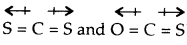both are linear molecules, but bond moments in CS2 cancel out so that the net dipole moment = O. But in OCS bond moment of C= O is not equal to that of C = S. Hence it has a net dipole moment. Thus dipole moment of OCS is higher.

Question 20.
Out of p-orbital and sp-hybrid orbital which has greater directional character and why?
sp orbital has a greater directional character than p-orbital. This is because the p-orbital has equal-sized lobes with equal electron density in both the lobes whereas the sp-hybrid orbital has greater electron density on one side.

Question 21.
Interpret the non-linear shape of H2S and non-planar shape of PCl3 using VSEPR theory.
H2S
No. of electron pairs around S = $$\frac{6+2}{2}=\frac{8}{2}$$ = 4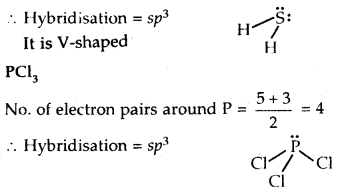Question 22.
Why mobility of H+ ions is greater in ice as compared to liquid water? [CBSE. PMT 2005]
H+ ions (obtained from ionization of water H2O) in liquid water get easily hydrated. As a result, the size of the hydrated H+ ions is much larger than in ice. Therefore the mobility of H+ ions in water is less than in ice.

Question 23.
What is the hybrid state of BeCl2? What will be the change in the hybrid state of BeCl2 in the solid-state? [CBSE PMT 2005]
In the vapor state at high temperature, BeCl2 exists as a linear molecule. Cl – Be – Cl due to sp hybridization of Be.

In the solid-state, it has a polymeric structure as follows:It is possible only due to the sp3 hybrid state of Be. Two half-filled hybrid orbitals will form normal covalent bonds with two Cl atoms. The other two Cl atoms are coordinated to Be atom by donating electron pairs into the empty hybrid orbitals of Be.

Question 24.
In both water and diethyl ether, the central atom viz. O- atom has the same hybridization. Then why do they have different bond angles? Which one has a greater bond angle?
Both water and diethyl ether have the central atom O in an sp3 hybrid state with two lone pairs of electrons on O.But due to the greater repulsions between two ethyl (C2H5) groups in diethyl ether than between two H-atoms in H2O result in a greater bond angle (110°) in diethyl ether than 104.5° in that of water (H2O).

Question 25.
BCl3 is planar but anhydrous AlCl3 is tetrahedral. Explain.
B atom in BCl3 is sp2 hybridized due to which the shape of BCl3 is triangular planar. Anhydrous AlCl3 exists as a dimer with the formula Al2Cl6.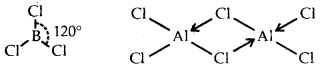Thus, each atom of A1 in Al2Cl6 is surrounded by four bond pairs and hence it assumes a tetrahedral structure.

Question 26.
(a) How bond energy varies from N2 to N2+ and why?
Both N2 and N2+ is, almost the same [though N2is slightly less stable and hence has less bond energy than N2+ due to the presence of a greater number of electrons in the antibonding molecular orbitals]

(b) On the basis of molecular orbital theory, what is a similarity between
(i) F2, O2
Both F2 and O2 have the same bond order = 1 and so the same bond length

(ii) CO, N2, NO+? [CBSE PMT 2004]
All of them have the same bond order and bond length.

Question 27.
Phosphorus pentachloride dissociates in the vapor phase as
PCl5 ⇌ PCl4+ + Cl
The hybridization of P in PCl5 is sp3d. What is it in PCl4+?
Phosphorus is sp3 hybridized in PCl4 since it is isostructural with NH4 which also has sp3 hybridization of Nitrogen.

Question 28.
Explain giving reasons whether BH4 and H3O+ will have the same/different geometry?
The central atom in both the ions is surrounded by the same number of pairs of electrons, that is, 4. Hence they have the same tetrahedral geometry.

Question 29.
State with reasons, which is more polar CO2 or N2O?
N2O is more polar than CO2 which is a linear molecule and thus symmetrical. Its net dipole moment is zero.N2O is linear but unsymmetrical. It is a resonance hybrid of the following canonical structures: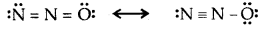It has a net dipole moment of 0.116 D.

Question 30.
Out of peroxide ion (O2) and superoxide ion (O2 ) which has larger bond length and why?
The bond order of O2 is 1.5 while that of O22- is 1.0.
The lesser the bond order, the greater is the bond length as the bond order is inversely proportional to bond length. ( Hence O22- has a larger bond length than O22-.

### Chemical Bonding and Molecular Structure Important Extra Questions Long Answer Type

Question 1.
Explain the formation of the following molecules according to the orbital concept, F2, HF, O2, H2O, N2, NH3 molecules.
1. Formation of F2 molecule. Atomic number (Z) of fluorine is 9 and its orbital electronic configuration is 1s2 2s2 2p2x, 2p2y, 2p1z. Thus, a fluorine atom has one half-filled atomic orbital. Therefore, two atoms of fluorine combine to form the fluorine molecule as a result of the combination for their half-filled atomic orbitals shown in Fig. The two atoms get linked by a single covalent bond.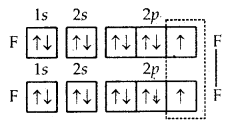Formation of F2 molecule

2. Formation of HF molecule. Fluorine atom, as stated above, has one half-filled atomic orbital. Hydrogen atom (Z = 1) has only one electron in Is orbital. Thus, the hydrogen fluoride (HF) molecule. is formed as a result of the combination (or overlap) of the half-filled orbitals belonging to the participating atoms.Formation of HF molecule

3. Formation of O2 molecules. The atomic number (Z) of oxygen is 8 and its orbital electronic configuration is 1s2 2s2 2p2x 2p1x 2p1z. This means that an oxygen atom has two half-filled orbitals with one electron each. Two such atoms will combine to form a molecule of oxygen as a result of the overlap of the half-filled orbitals with opposite spins of electrons.Formation of O2 molecule
Thus, the two atoms of oxygen are bonded to each other by two covalent bonds or double bonds (O = O).

4. Formation of H2O molecule. In the formation of the H2O molecule, the two half-filled orbitals of the oxygen atom combine with the half-filled orbitals (1s) of the hydrogen atoms. Thus, the oxygen atom gets linked to the two hydrogen atoms by single covalent bonds as shown in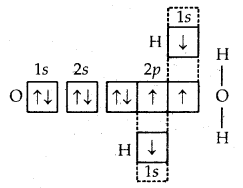Formation of H2O molecule

5. Formation of N2 molecule. The atomic number of nitrogen is 7 and its orbital electronic configuration is 1s2 2s2 2p1x 2p1y 2p1 z. This shows that the nitrogen atom has three half-filled atomic orbitals. Two such atoms combine as a result of the overlap of the three half-filled orbitals and a triple bond gets formed (N = N).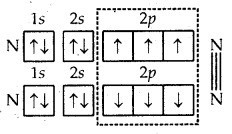Formation of N2 molecule

6. Formation of NH3 molecule. In the formation of ammonia (NH3) molecule, three half-filled orbitals present in the valence shell of nitrogen atom combine with 1s orbital of three hydrogen atoms with one electron each. As a result, the nitrogen atom completes its octet and a molecule of NH3 is formed in which the nitrogen atom is linked to three hydrogen atoms by covalent bonds.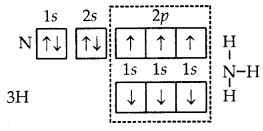Formation of NH3 molecule

Question 2.
What is a hydrogen bond, what are its causes, and give the conditions for hydrogen bonding? What is the strength of hydrogen bonding? Describe the two types of hydrogen bonding.
When hydrogen is connected to small highly electronegative atoms such as F, O, and N in such cases hydrogen forms an electrostatic weak bond with an electronegative atom of the second molecule, this type of bond binds the hydrogen atom of one molecule and the electronegative atom of the 2nd molecule is called as hydrogen bond. It is a weak bond and it is denoted by dotted lines e.g., in HF, hydrogen forms a weak bond with the electronegative F atom of the 2nd molecule neighboring HF.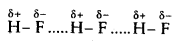So it means hydrogen is acting as a bridge between two molecules by one covalent bond and the other by a hydrogen bond. Due to this hydrogen bonding, HF will not exist as a single molecule but it will exist as an associated molecule (HF)n. So hydrogen bond may be defined as a weak electrostatic bond that binds the hydrogen atom of one molecule and electronegative bond atoms (F, O, N) of the second neighboring molecule.

Cause of hydrogen bonding: When a hydrogen atom is bonded to an electronegative atom (say F, O, N) through a covalent bond, due to electronegativity difference, the electronegative atom attracts the shared pair of electrons towards its side with a great force as a result of which the shared pair of electrons will be displaced toward electronegative atom and away from a hydrogen atom.

Due to which hydrogen atom will acquire a slightly negative charge and if another molecule is brought nearer to it in such a way that electronegative atom of the second molecule faces hydrogen atom of the 1st molecule, due to opposite charges present on the atoms, an electrostatic bond will be formed between the hydrogen atom of one molecule and electronegative atom of 2nd molecule and this is called as hydrogen bond.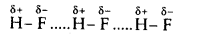Conditions for hydrogen bonding. The following two necessary conditions for hydrogen bonding are:

1. Hydrogen atom should be connected to highly electronegative atom say F, O, or N.
2. The electronegative atom of which the hydrogen atom is connected should be the same in size.

The smaller the size of the electronegative atom greater will be the attraction of that atom for shared pair of electrons and hence that pair will be displaced more nearer to that atom and hence that atom will develop greater negative charge and the hydrogen atom will develop a greater positive charge and hence hydrogen atom of this molecule will easily attract negative atom of the IInd molecule and hence a hydrogen bond will be easily formed.

As both these conditions are satisfied only by F, O, N atoms so only three atoms show hydrogen bond.

Strength of Hydrogen Bond: A hydrogen bond is a very weak bond. It is weaker than an ionic or a covalent bond. Its strength range from 13 kJ mol-1 to 42 kJ mol-1. The strength of the hydrogen bond for some of the molecules is the order H-F H (40 kJ mol-1) > O-H…… O (28 kJ mol-1) > H-N…… H (13 kJ mol-1) whereas the strength of a covalent bond is quite high. For example, the bond strength of the H-H bond in H2 is 433 kJ mol-1.

Types of H-bonding
There are two types of hydrogen-bonds

1. Intermolecular hydrogen bond. It is formed between two different molecules of the same or different compounds. For example H-bond in case of HF, alcohol, or water.
2. Intramolecular Hydrogen bond. It is formed when a hydrogen atom is in between the two highly electronegative (F, O, N) atoms present within the same molecule. For example, in o-nitrophenol, hydrogen is in between the two oxygen atoms.Question 3.
Compare the properties of electrovalent and covalent compounds.
 Electrovalent/Ionic compounds Covalent compounds 1. They are formed by sharing of electrons between two atoms.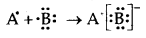1. They are formed by sharing of electrons between two atoms.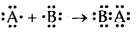2. These compounds may be solids, liquids, or gases. 2. These compounds may be solids, liquids, or gases. 3. They are made up of molecules held together by weak van der Waals forces 3. They are made up of molecules held together by weak van der Waals forces of attraction. 4. They have generally high M and B points 4.            They have generally low melting and boiling points. 5. They are generally soluble in polar solvents like water and insoluble in organic solvents. 5. Covalent compounds are generally soluble in non-polar solvents such as benzene and insoluble in polar solvents. 6. Ionic compounds conduct electricity in the molten or dissolved state. 6. Covalent compounds are generally bad conductors of electricity. 7. Ionic compounds are non-directional and do not show isomerism. 7. Covalent compounds are rigid and directional. They show isomerism. 8. These compounds undergo ionic reactions which are very fast, almost instantaneous. 8. These compounds undergo molecular reactions which are very slow.